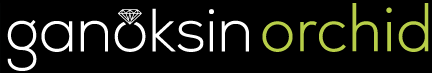# Qbasic Program

Here is the first of the Qbasic programs. More to follow. Feedback
welcomed. I added the GemNet list to my addressees at the suggestion of
Clive Billing (thanks, Clive). As I said before, this is public domain:
give away free to everybody, don’t sell to anybody.

SCREEN 11: DEFSNG A-Z
RingMover = 0
Stepper = .043
InterMultX = 3
InterMultY = 3.3
BoxMult = .11
X = -30
Y1 = 190
Y2 = 260
10 RingMover = RingMover + Stepper
X = X + InterMultX * ABS(COS(RingMover))
Y1 = Y1 + InterMultY * SIN(RingMover)
Y2 = Y2 - InterMultY * SIN(RingMover)
EndX = X + Y1 * BoxMult
EndY1 = Y1 + Y1 * BoxMult
EndY2 = Y2 + Y2 * BoxMult
LINE (X, Y1)-(EndX, EndY1), 0, BF
LINE (X, Y1)-(EndX, EndY1), 1, B
LINE (X, Y2)-(EndX, EndY2), 0, BF
LINE (X, Y2)-(EndX, EndY2), 1, B
20 IF X < 700 THEN GOTO 10 ELSE GOTO 20

Joel Kahn <@Joel_Kahn>
Comptroller for Maxon’s Jewelers
Diamond Merchants & Estate Jewelers
Authorized Dealer for Rolex & Tag Heuer
2622 S Glenstone, Springfield Missouri 65804 USA
Voice: 417-887-1800 or 417-887-1809
Fax: 417-887-3422

More source code:

SCREEN 11: DEFSNG A-Z
M = 0
S = .023
X1 = 260
Ystart = 5
Y1 = Ystart
XA = 4.8
YA = 2.6
10 M = M + S
X1 = X1 + XA * COS(M)
Y1 = Y1 + YA * SIN(M)
B = ABS(Y1 - Ystart) / 4
X2 = X1 + B
Y2 = Y1 + B
LINE (X1, Y1)-(X2, Y2), 0, BF
LINE (X1, Y1)-(X2, Y2), 1, B
X3 = X2 + B
Y3 = Y2 + B
LINE (X2, Y2)-(X3, Y3), 0, BF
LINE (X2, Y2)-(X3, Y3), 1, B
X4 = X3 + B
Y4 = Y3 + B
LINE (X3, Y3)-(X4, Y4), 0, BF
LINE (X3, Y3)-(X4, Y4), 1, B
X5 = X4 + B
Y5 = Y4 + B
LINE (X4, Y4)-(X5, Y5), 0, BF
LINE (X4, Y4)-(X5, Y5), 1, B
GOTO 10

Joel Kahn <@Joel_Kahn>
Comptroller for Maxon’s Jewelers
Diamond Merchants & Estate Jewelers
Authorized Dealer for Rolex & Tag Heuer
2622 S Glenstone, Springfield Missouri 65804 USA
Voice: 417-887-1800 or 417-887-1809
Fax: 417-887-3422